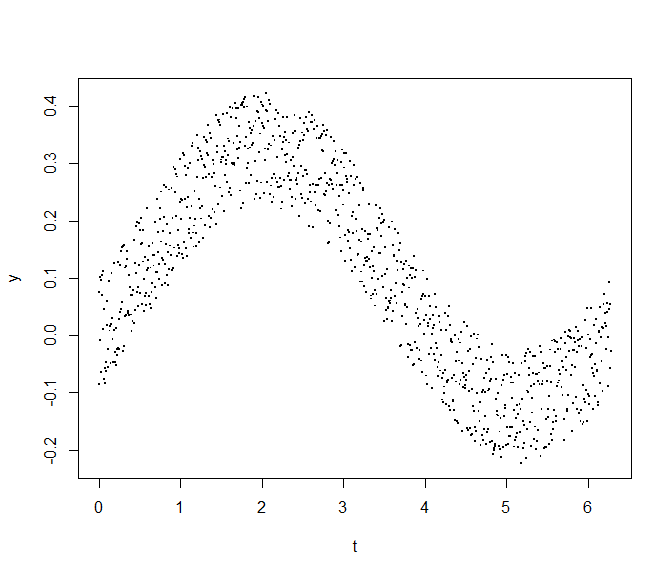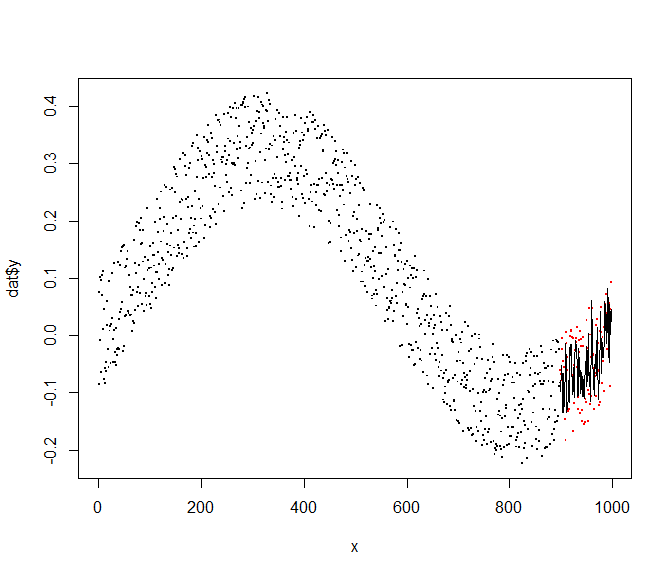# Using ‘splitTools’

## Introduction

{splitTools} is a fast, lightweight toolkit for data splitting.

Its two main functions partition() and create_folds() support

• data partitioning (e.g. into training, validation and test),

• creating folds for cross-validation (CV),

• creating repeated folds for CV,

• stratified splitting (e.g. for stratified CV),

• grouped splitting (e.g. for group-k-fold CV) as well as

• blocked splitting (if the sequential order of the data should be retained).

The function create_timefolds() does time-series splitting in the sense that the out-of-sample data follows the in-sample data.

We will now illustrate how to use {splitTools} in a typical modeling workflow.

## Data partitioning

We will go through the following steps:

1. We split the iris data into 60% training, 20% validation, and 20% test data, stratified by the variable Sepal.Length. Since this variable is numeric, stratification uses quantile binning.

2. Based on the validation performance, we tune the parameter mtry of a random forest with response Sepal.Length.

3. After selecting the best mtry, we evaluate the final model on the test data.

library(splitTools)
library(ranger)

# Split data into partitions
set.seed(3451)
inds <- partition(iris$Sepal.Length, p = c(train = 0.6, valid = 0.2, test = 0.2)) str(inds) #> List of 3 #>$ train: int [1:81] 2 3 6 7 8 10 11 18 19 20 ...
#>  $valid: int [1:34] 1 12 14 15 27 34 36 38 42 48 ... #>$ test : int [1:35] 4 5 9 13 16 17 25 39 41 45 ...

train <- iris[inds$train, ] valid <- iris[inds$valid, ]
test <- iris[inds$test, ] # Root-mean-squared error function used to evaluate results rmse <- function(y, pred) { sqrt(mean((y - pred)^2)) } # Tune mtry on validation data valid_mtry <- numeric(ncol(train) - 1) for (i in seq_along(valid_mtry)) { fit <- ranger(Sepal.Length ~ ., data = train, mtry = i) valid_mtry[i] <- rmse(valid$Sepal.Length, predict(fit, valid)$predictions) } valid_mtry #>  0.3809234 0.3242173 0.3208119 0.3241518 (best_mtry <- which.min(valid_mtry)) #>  3 # Fit and test final model final_fit <- ranger(Sepal.Length ~ ., data = train, mtry = best_mtry) rmse(test$Sepal.Length, predict(final_fit, test)$predictions) #>  0.3480947 ## CV Since the iris data consists of only 150 rows, investing 20% of observations for validation seems like a waste of resources, and the performance estimates might not be very robust. So let’s replace simple validation by five-fold CV, again using stratification on the response variable. 1. Split iris into 80% training data and 20% test, stratified by the variable Sepal.Length. 2. Use stratified five-fold CV to tune the parameter mtry. 3. After selecting the best mtry by this “GridSearchCV”, we evaluate the final model on the test data. # Split into training and test inds <- partition(iris$Sepal.Length, p = c(train = 0.8, test = 0.2))

train <- iris[inds$train, ] test <- iris[inds$test, ]

# Get stratified CV in-sample indices
folds <- create_folds(train$Sepal.Length, k = 5) # Tune mtry by GridSearchCV valid_mtry <- numeric(ncol(train) - 1) for (i in seq_along(valid_mtry)) { cv_mtry <- numeric() for (fold in folds) { fit <- ranger(Sepal.Length ~ ., data = train[fold, ], mtry = i) cv_mtry <- c( cv_mtry, rmse(train[-fold, "Sepal.Length"], predict(fit, train[-fold, ])$predictions)
)
}
valid_mtry[i] <- mean(cv_mtry)
}

# Result of CV
valid_mtry
#>  0.3870915 0.3460605 0.3337763 0.3302831
(best_mtry <- which.min(valid_mtry))
#>  4

# Use optimal mtry to make model
final_fit <- ranger(Sepal.Length ~ ., data = train, mtry = best_mtry)
rmse(test$Sepal.Length, predict(final_fit, test)$predictions)
#>  0.2970876

## Repeated CV

If feasible, repeated CV is recommended in order to reduce uncertainty in decisions. Otherwise, the process remains the same. When we use three repetitions in 5-fold CV, instead of getting five performance estimates, we get 15 such values.

# We start by making repeated, stratified CV folds
folds <- create_folds(train$Sepal.Length, k = 5, m_rep = 3) length(folds) #>  15 for (i in seq_along(valid_mtry)) { cv_mtry <- numeric() for (fold in folds) { fit <- ranger(Sepal.Length ~ ., data = train[fold, ], mtry = i) cv_mtry <- c( cv_mtry, rmse(train[-fold, "Sepal.Length"], predict(fit, train[-fold, ])$predictions)
)
}
valid_mtry[i] <- mean(cv_mtry)
}

# Result of CV
valid_mtry
#>  0.3934294 0.3544207 0.3422013 0.3393454
(best_mtry <- which.min(valid_mtry))
#>  4

# Use optimal mtry to make model
final_fit <- ranger(Sepal.Length ~ ., data = train, mtry = best_mtry)
rmse(test$Sepal.Length, predict(final_fit, test)$predictions)
#>  0.2937055

## Time-series CV and block partitioning

When modeling time series, usual CV destroys the sequential nature of the data. This can be avoided by the following modification of k-fold CV:

The data is first split into $$k+1$$ blocks $$B_1, ..., B_{k+1}$$, in sequential order. Depending of type = "extending" (default) or type == "moving", the following data sets are used in CV:

• First fold: $$B_1$$ is used for training, $$B_2$$ for evaluation.

• Second fold: $$\{B_1, B_2\}$$ (type “extending”) or $$B_2$$ (type “moving”) are used for training, $$B_3$$ for evaluation.

• $$k$$-th fold: Rows in $$\{B_1, ..., B_k\}$$ (“extending”) or $$B_{k}$$(“moving”) are used for training, those in $$B_{k+1}$$ for evaluation.

These schemata make sure that the evaluation data always follows the training data. Note that the training data grows over the whole process linearly with type = "extending", whereas its length is approximately constant with type = "moving".

In order to have a final evaluation of the optimized model, typically an initial blocked split into sequential training and testing data is done.

### Example

We first create a time series and derive lagged features for training. Then, again, we optimize mtry of a random forest by time-series CV. We evaluate the optimized model on the last 10% of the time series.

# Create data
set.seed(452)
n <- 1000
t <- seq(0, 2 * pi, length.out = n)
y <- 0.2 * sin(t) - 0.1 * cos(t) + 0.2 * runif(n)
plot(y ~ t, pch = ".", cex = 2)# Helper function
Lag <- function(z, k = 1) {
c(z[-seq_len(k)], rep(NA, k))
}
Lag(1:4, k = 1)
#>   2  3  4 NA

dat <- data.frame(
y,
lag1 = Lag(y),
lag2 = Lag(y, k = 2),
lag3 = Lag(y, k = 3)
)
dat <- dat[complete.cases(dat), ]
#>              y         lag1         lag2        lag3
#> 1 -0.085447174  0.075314649 -0.007841658  0.10081516
#> 2  0.075314649 -0.007841658  0.100815165  0.09635395
#> 3 -0.007841658  0.100815165  0.096353945 -0.06476294
#> 4  0.100815165  0.096353945 -0.064762935  0.10474890
#> 5  0.096353945 -0.064762935  0.104748904  0.07030228
#> 6 -0.064762935  0.104748904  0.070302283  0.01085425
cor(dat)
#>              y      lag1      lag2      lag3
#> y    1.0000000 0.8789100 0.8858208 0.8840929
#> lag1 0.8789100 1.0000000 0.8791226 0.8858995
#> lag2 0.8858208 0.8791226 1.0000000 0.8791388
#> lag3 0.8840929 0.8858995 0.8791388 1.0000000

# Block partitioning
inds <- partition(dat$y, p = c(train = 0.9, test = 0.1), type = "blocked") str(inds) #> List of 2 #>$ train: int [1:898] 1 2 3 4 5 6 7 8 9 10 ...
#>  $test : int [1:99] 899 900 901 902 903 904 905 906 907 908 ... train <- dat[inds$train, ]
test <- dat[inds$test, ] # Get time series folds folds <- create_timefolds(train$y, k = 5)
str(folds)
#> List of 5
#>  $Fold1:List of 2 #> ..$ insample : int [1:150] 1 2 3 4 5 6 7 8 9 10 ...
#>   ..$outsample: int [1:150] 151 152 153 154 155 156 157 158 159 160 ... #>$ Fold2:List of 2
#>   ..$insample : int [1:300] 1 2 3 4 5 6 7 8 9 10 ... #> ..$ outsample: int [1:150] 301 302 303 304 305 306 307 308 309 310 ...
#>  $Fold3:List of 2 #> ..$ insample : int [1:450] 1 2 3 4 5 6 7 8 9 10 ...
#>   ..$outsample: int [1:150] 451 452 453 454 455 456 457 458 459 460 ... #>$ Fold4:List of 2
#>   ..$insample : int [1:600] 1 2 3 4 5 6 7 8 9 10 ... #> ..$ outsample: int [1:150] 601 602 603 604 605 606 607 608 609 610 ...
#>  $Fold5:List of 2 #> ..$ insample : int [1:750] 1 2 3 4 5 6 7 8 9 10 ...
#>   ..$outsample: int [1:148] 751 752 753 754 755 756 757 758 759 760 ... # Tune mtry by GridSearchCV valid_mtry <- numeric(ncol(train) - 1) for (i in seq_along(valid_mtry)) { cv_mtry <- numeric() for (fold in folds) { fit <- ranger(y ~ ., data = train[fold$insample, ], mtry = i)
cv_mtry <- c(
cv_mtry,
rmse(train[fold$outsample, "y"], predict(fit, train[fold$outsample, ])$predictions) ) } valid_mtry[i] <- mean(cv_mtry) } # Result of CV valid_mtry #>  0.08227426 0.08188822 0.08230784 (best_mtry <- which.min(valid_mtry)) #>  2 # Use optimal mtry to make model and evaluate on future test data final_fit <- ranger(y ~ ., data = train, mtry = best_mtry) test_pred <- predict(final_fit, test)$predictions
rmse(test$y, test_pred) #>  0.07184702 # Plot x <- seq_along(dat$y)
plot(x, dat$y, pch = ".", cex = 2) points(tail(x, length(test$y)), test$y, col = "red", pch = ".", cex = 2) lines(tail(x, length(test$y)), test_pred)## Stratification on multiple columns

The function multi_strata() creates a stratification factor from multiple columns that can then be passed to create_folds(, type = "stratified") or partition(, type = "stratified"). The resulting partitions will be (quite) balanced regarding these columns.

Two grouping strategies are offered:

1. k-means clustering based on scaled input.
2. All combinations of columns, where numeric input is being binned.

Let’s have a look at a simple example where we want to model “Sepal.Width” as a function of the other variables in the iris data set. We want to do a stratified train/valid/test split, aiming at being balanced regarding not only the response “Sepal.Width”, but also regarding the important predictor “Species”. In this case, we could use the following workflow:

set.seed(3451)

ir <- iris[c("Sepal.Length", "Species")]
y <- multi_strata(ir, k = 5)
inds <- partition(
y, p = c(train = 0.6, valid = 0.2, test = 0.2), split_into_list = FALSE
)

# Check
by(ir, inds, summary)
#> inds: train
#>   Sepal.Length         Species
#>  Min.   :4.300   setosa    :30
#>  1st Qu.:5.100   versicolor:30
#>  Median :5.800   virginica :30
#>  Mean   :5.836
#>  3rd Qu.:6.400
#>  Max.   :7.700
#> ------------------------------------------------------------
#> inds: valid
#>   Sepal.Length         Species
#>  Min.   :4.400   setosa    :10
#>  1st Qu.:5.425   versicolor:10
#>  Median :5.900   virginica :10
#>  Mean   :5.903
#>  3rd Qu.:6.300
#>  Max.   :7.900
#> ------------------------------------------------------------
#> inds: test
#>   Sepal.Length         Species
#>  Min.   :4.700   setosa    :10
#>  1st Qu.:5.100   versicolor:10
#>  Median :5.700   virginica :10
#>  Mean   :5.807
#>  3rd Qu.:6.475
#>  Max.   :7.100